# NCERT Exemplar Class 11 Physics Solutions for Chapter 7 - System Of Particles And Rotational Motion

NCERT Exemplar Class 11 Physics Chapter 7 System of Particles and Rotational Motion is a study material that consists of advanced level questions that are usually asked in engineering/medical entrance examination and competitive examination. To understand the topic clearly, students have to solve and get familiar with NCERT exemplar Class 11 Physics Chapter 7.
NCERT exemplar class 11 physics Chapter 7 consists of questions provided in NCERT exemplar book together with the system of particles and rotational motion important questions, rotational motion important questions NEET, problems on the system of particles, MCQs, worksheets and exercises. The system of particles and rotational motion deals with the system of particles and the arrangement in which they form. The rotational motion of particles is primarily concerned with the way different particles move. Some of the other major concepts that are introduced in this chapter are:

1. Centre of Mass
2. The motion of Centre of Mass
3. Rolling Motion
4. Angular Velocity
5. Product of Vectors
6. Equilibrium of a rigid body
7. Kinematics and Dynamics of rotational motion
8. Angular Momentum

## Download the PDF of NCERT Exemplar Class 11 Physics Chapter 7 System of Particles and Rotational Motion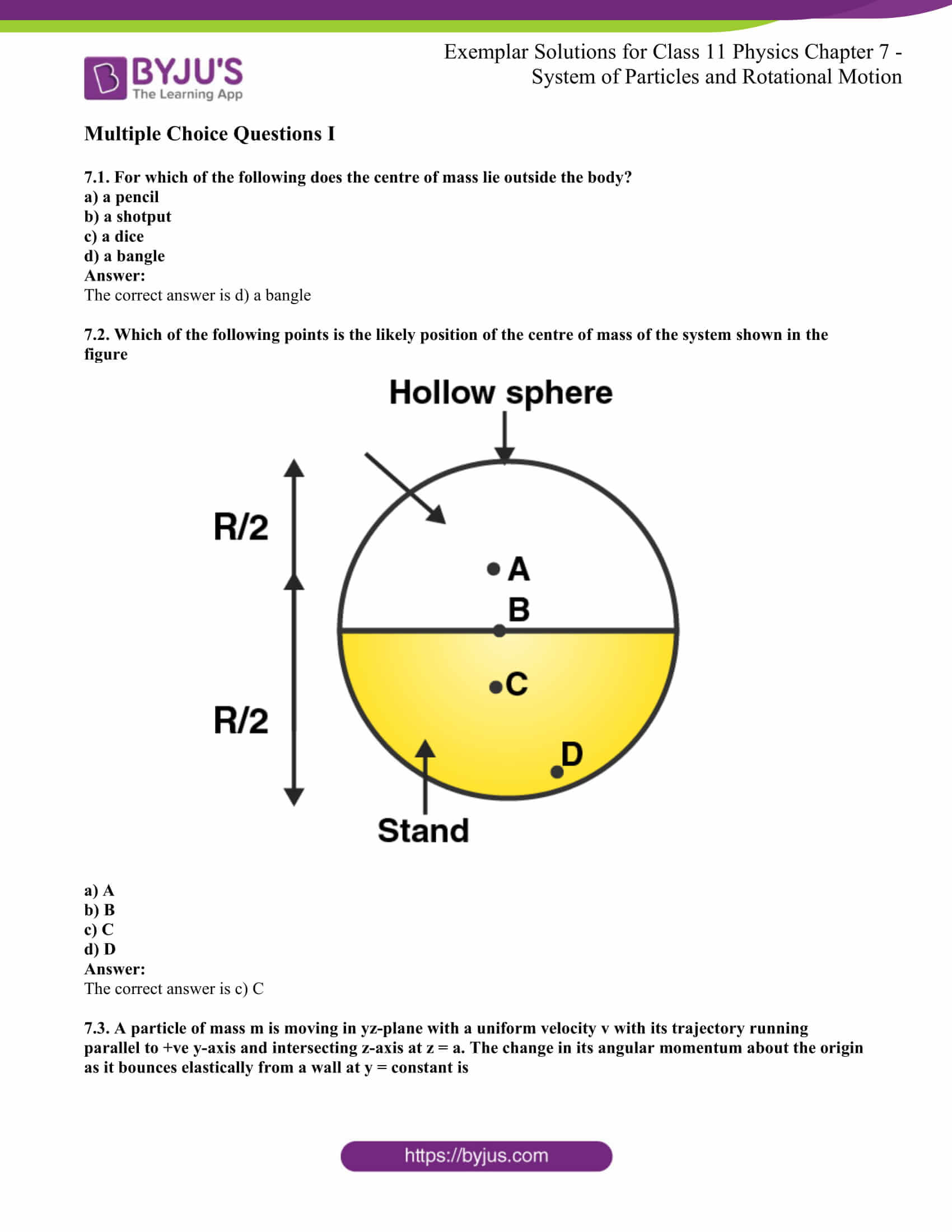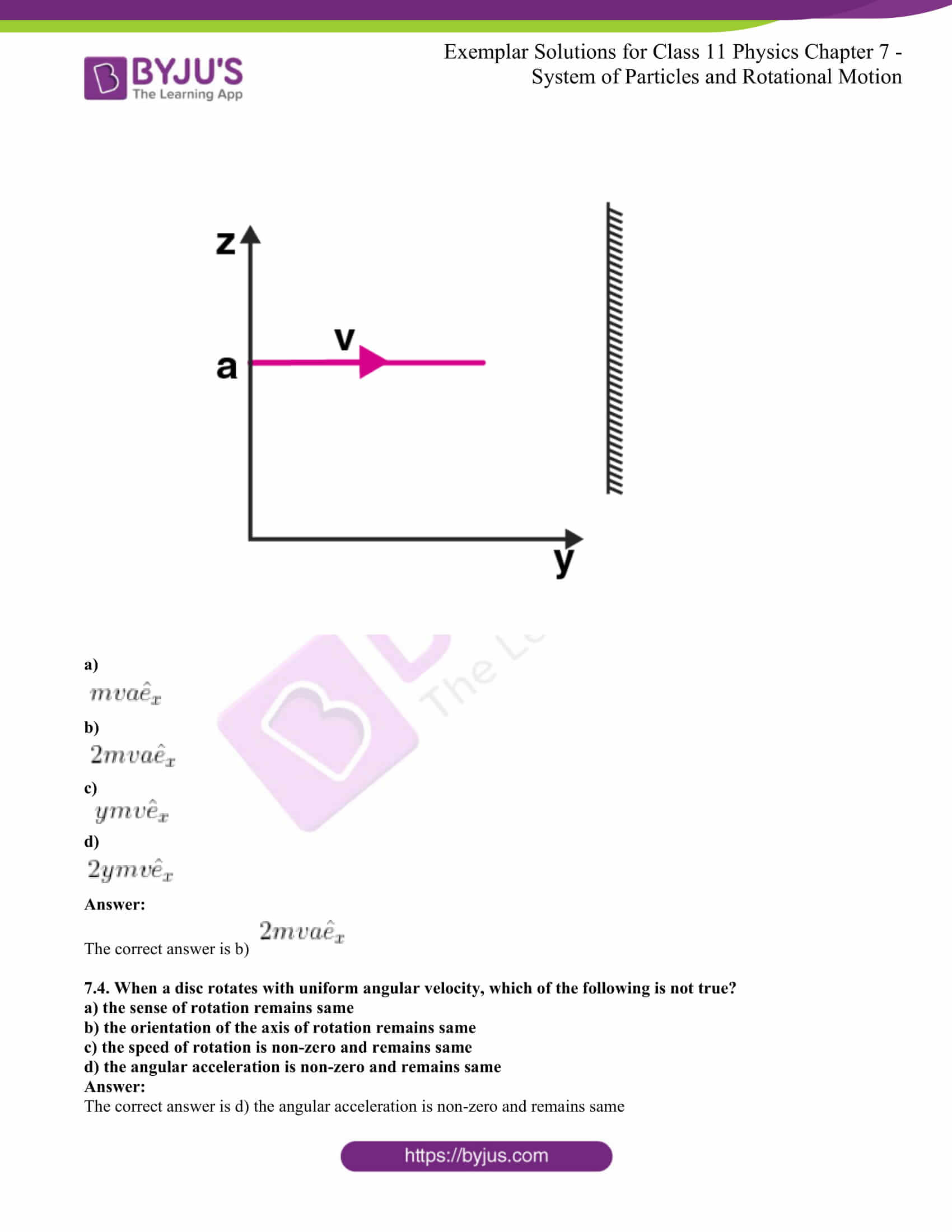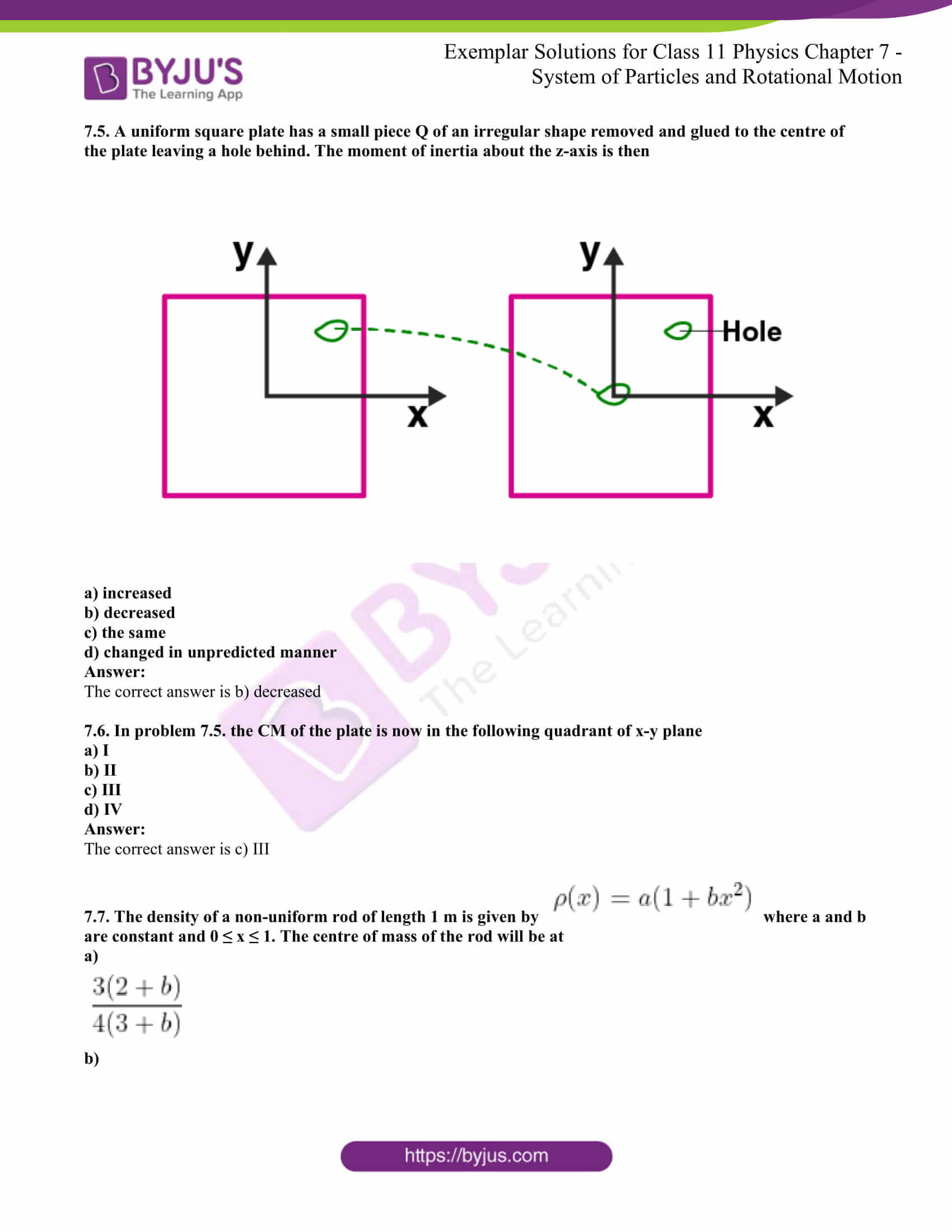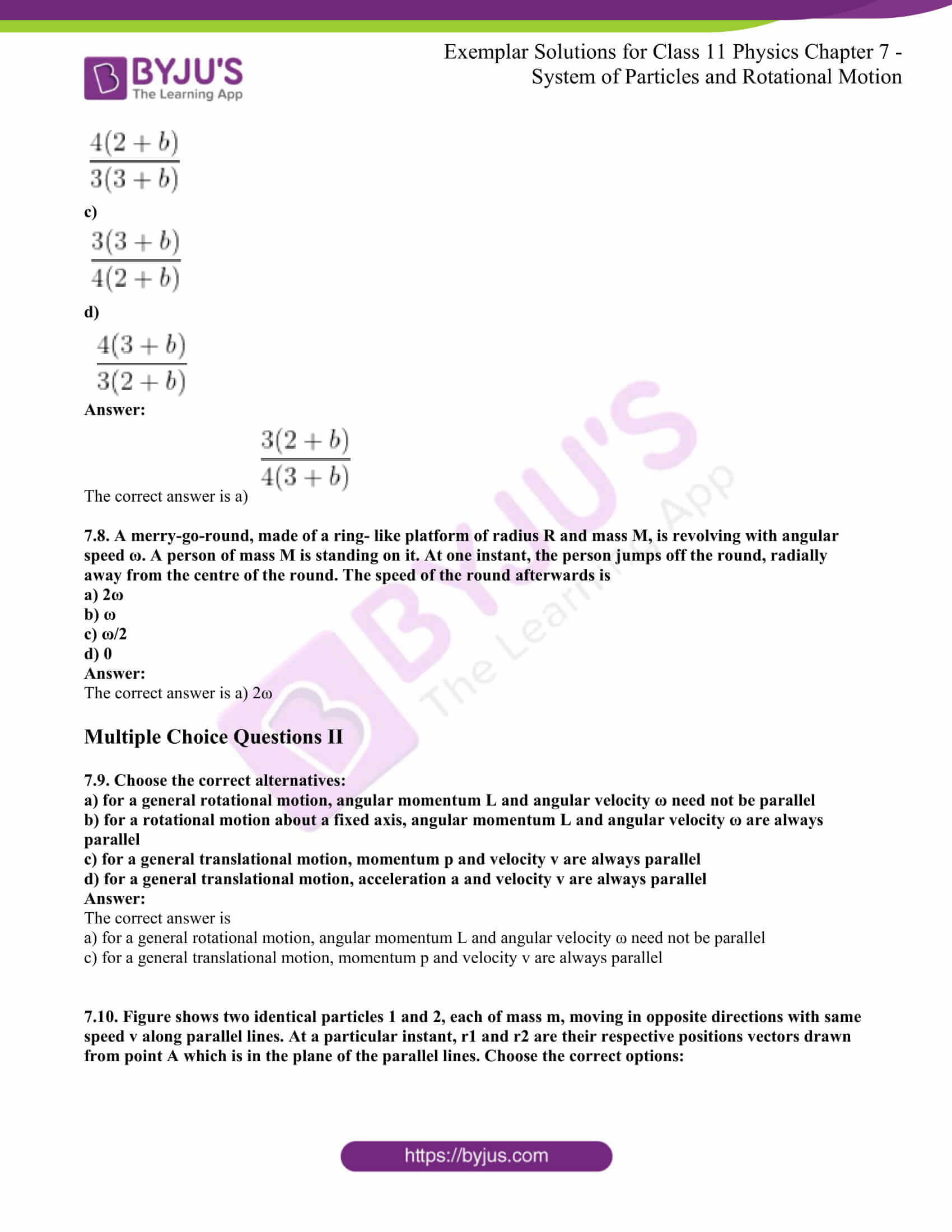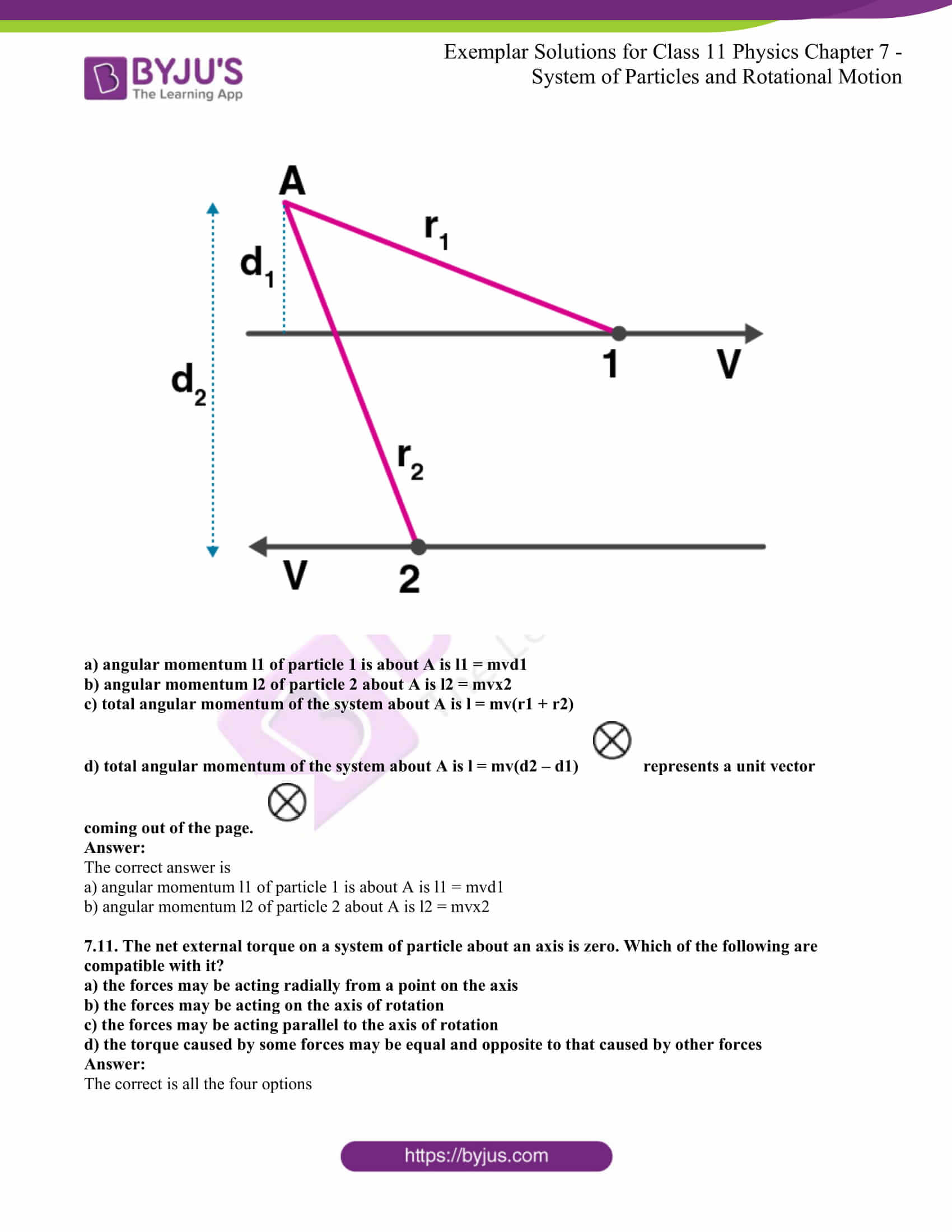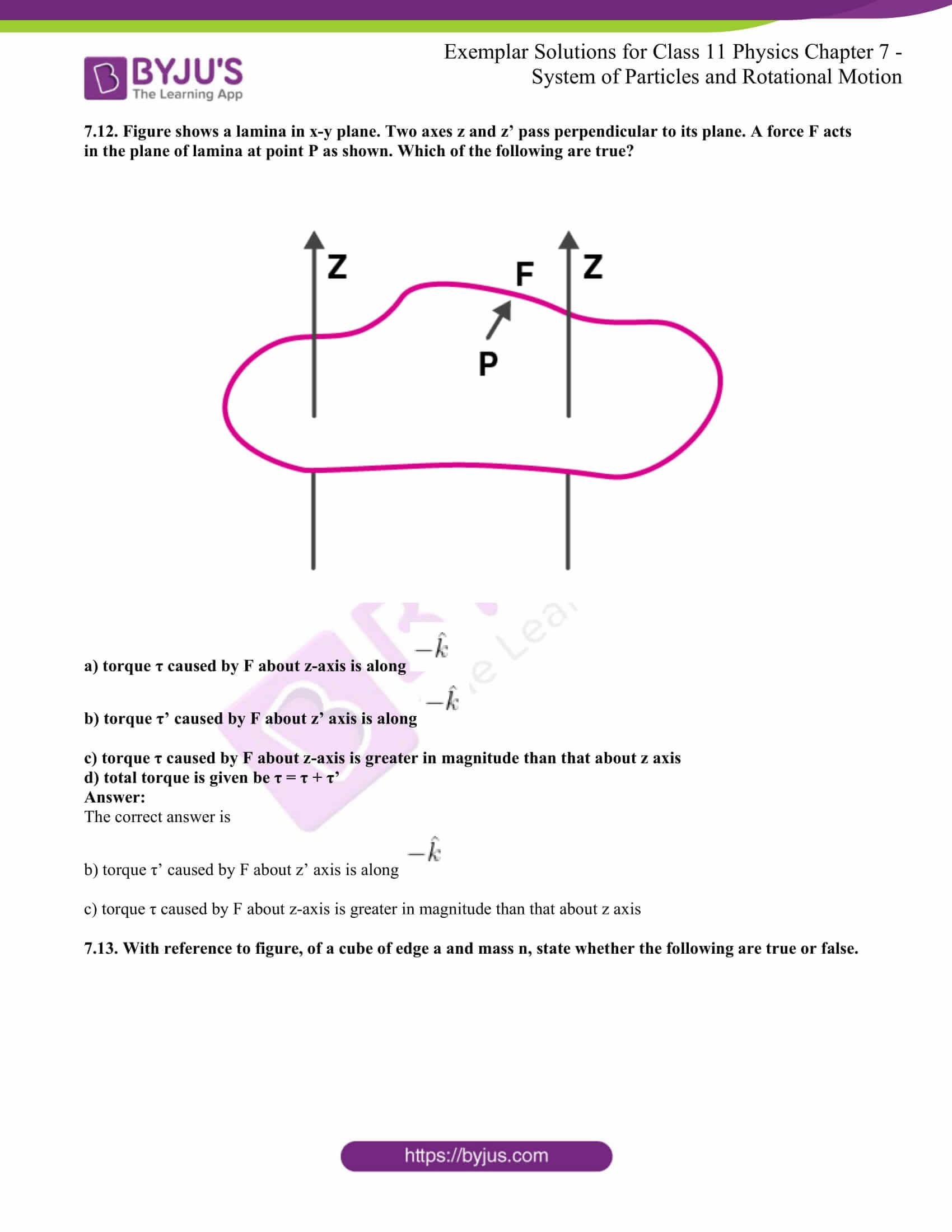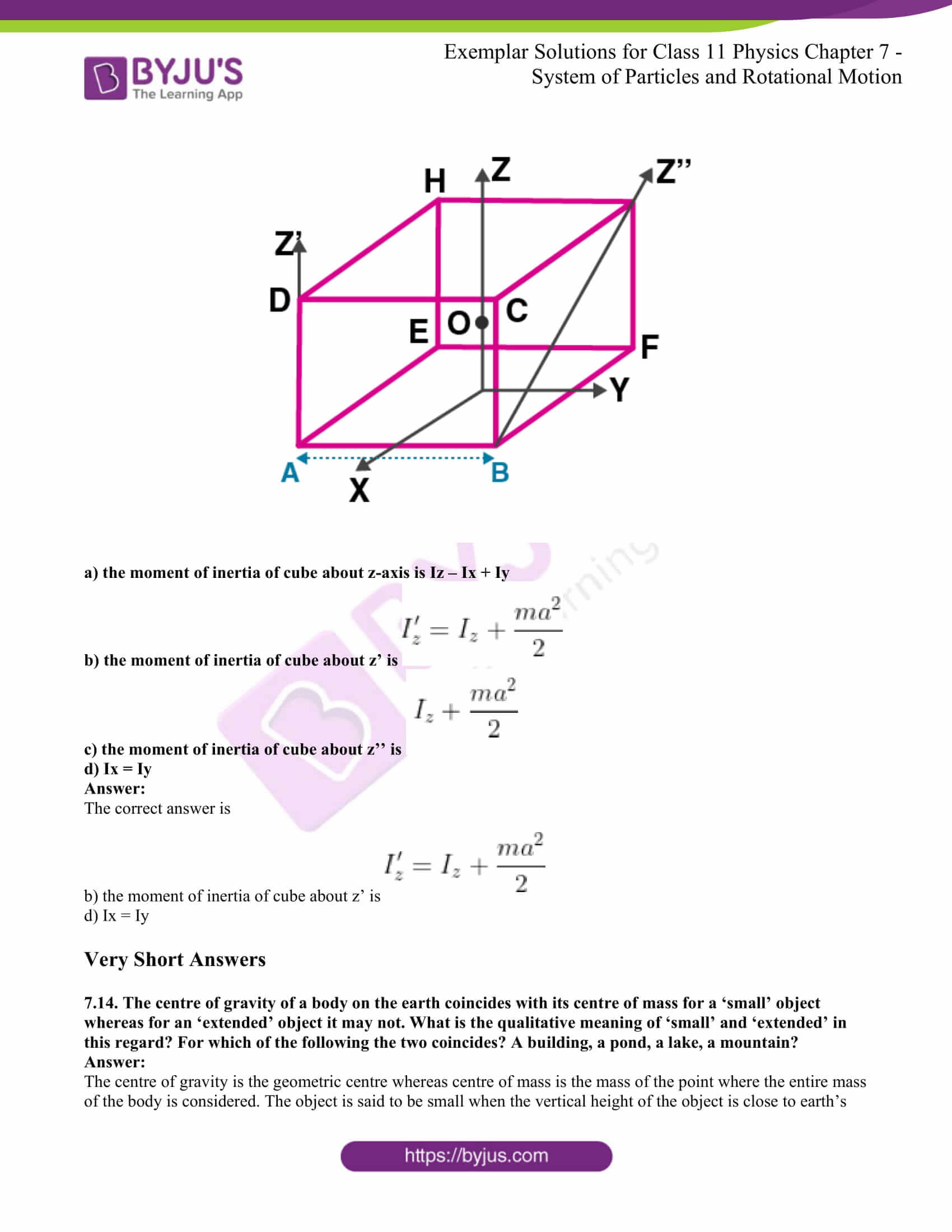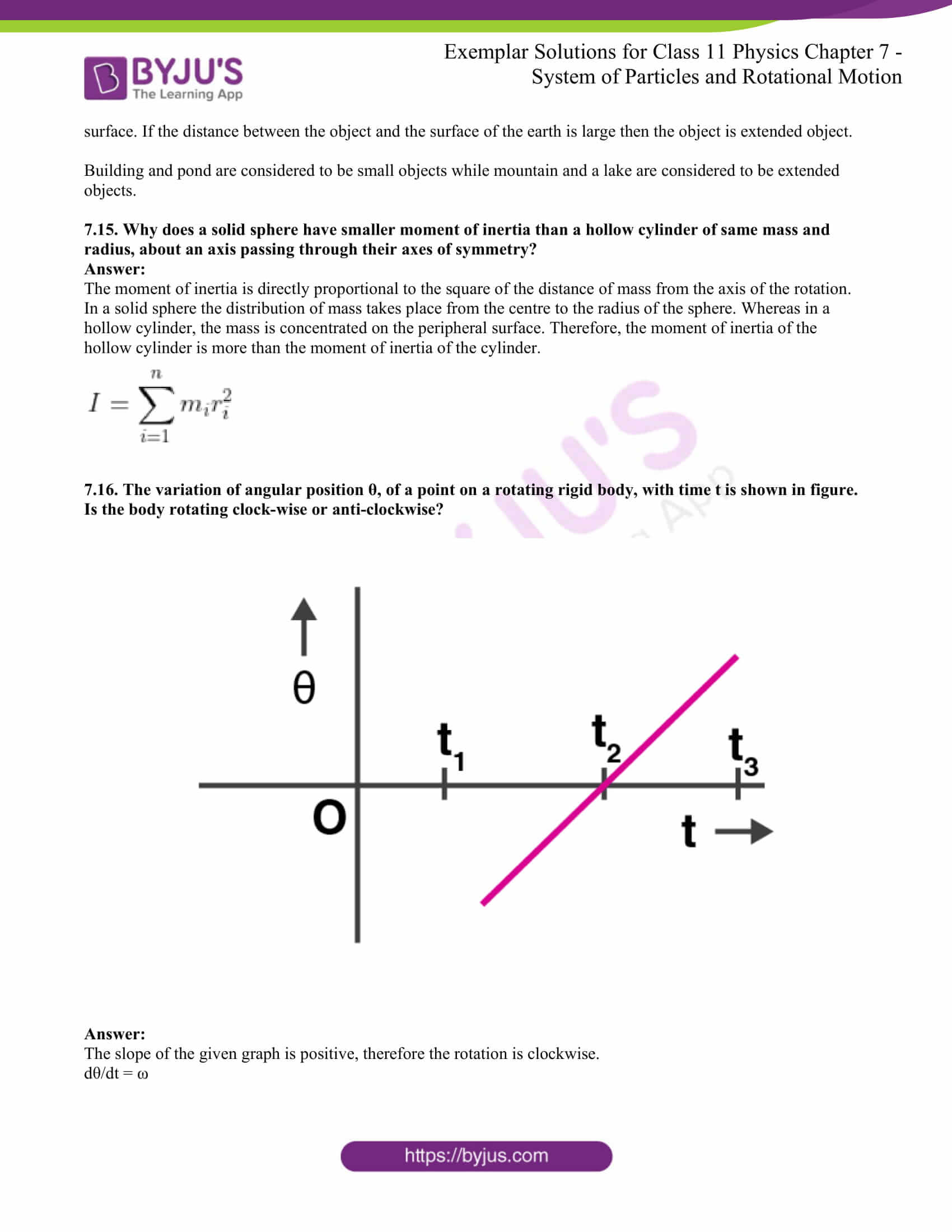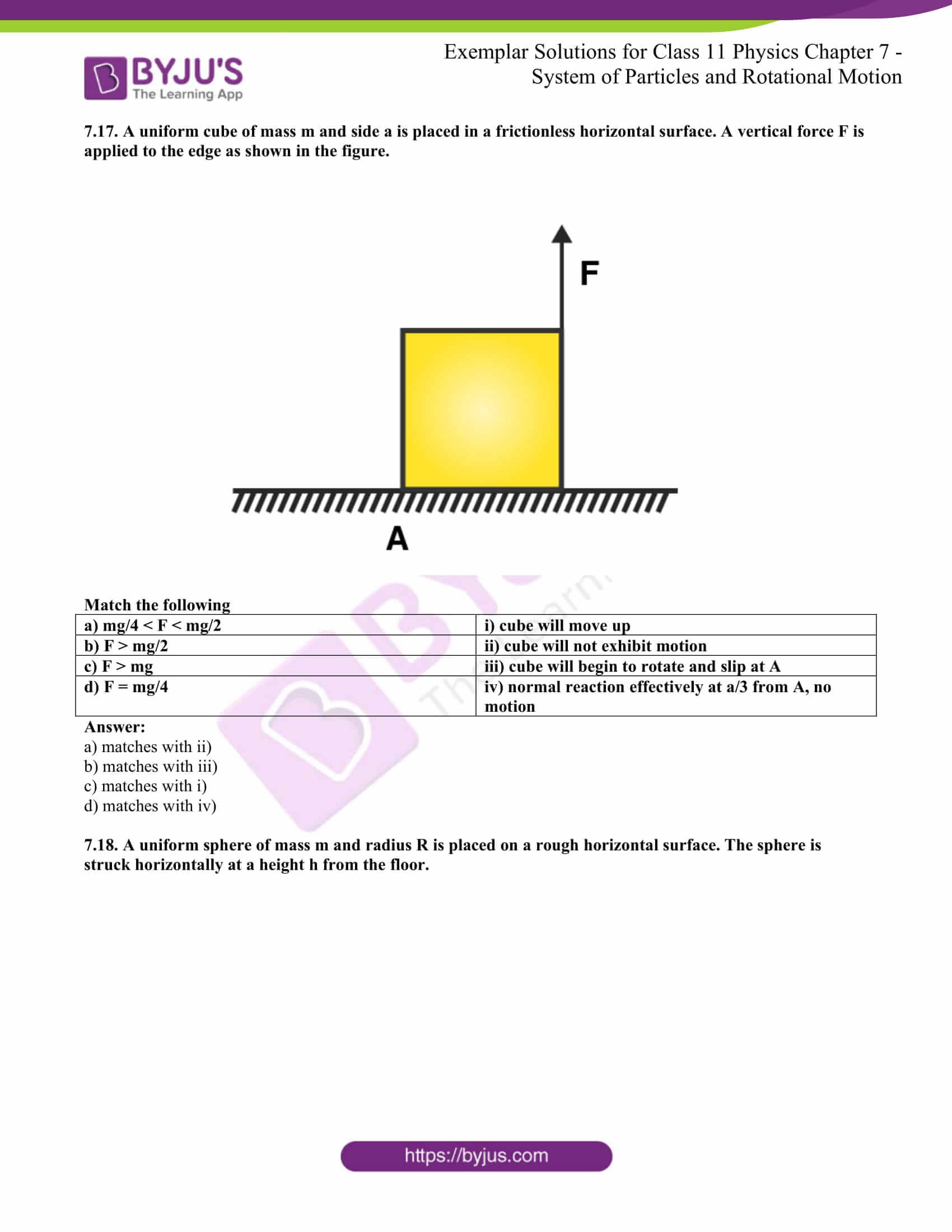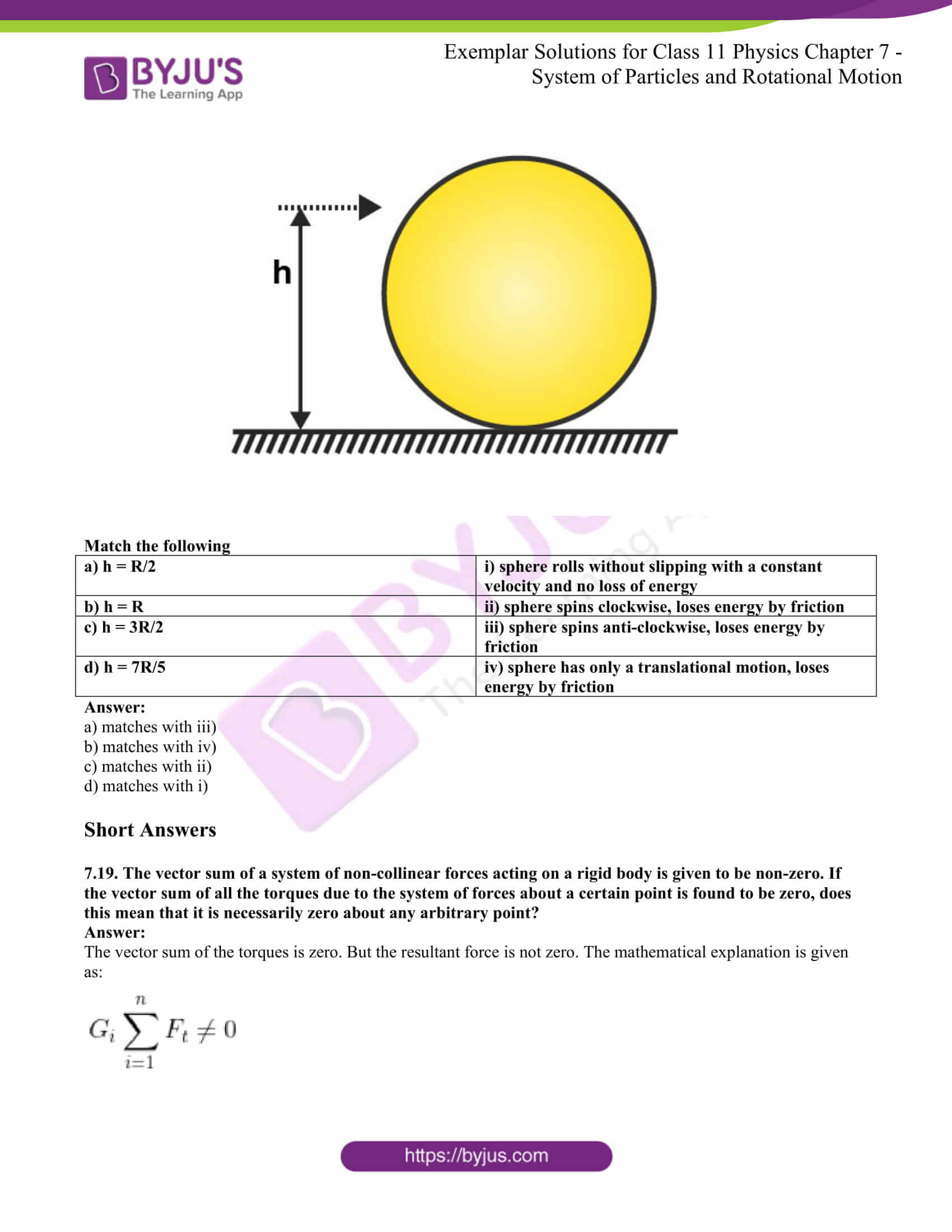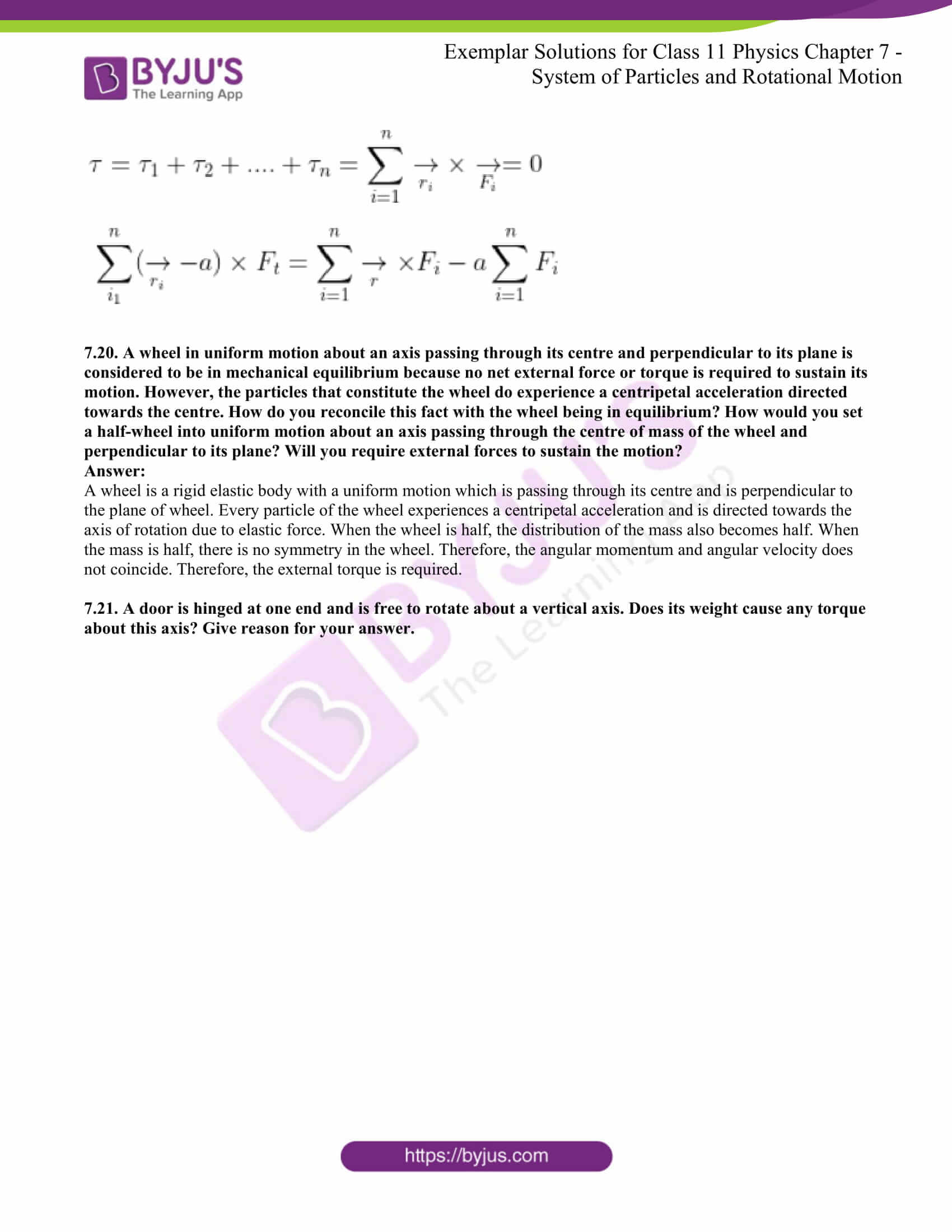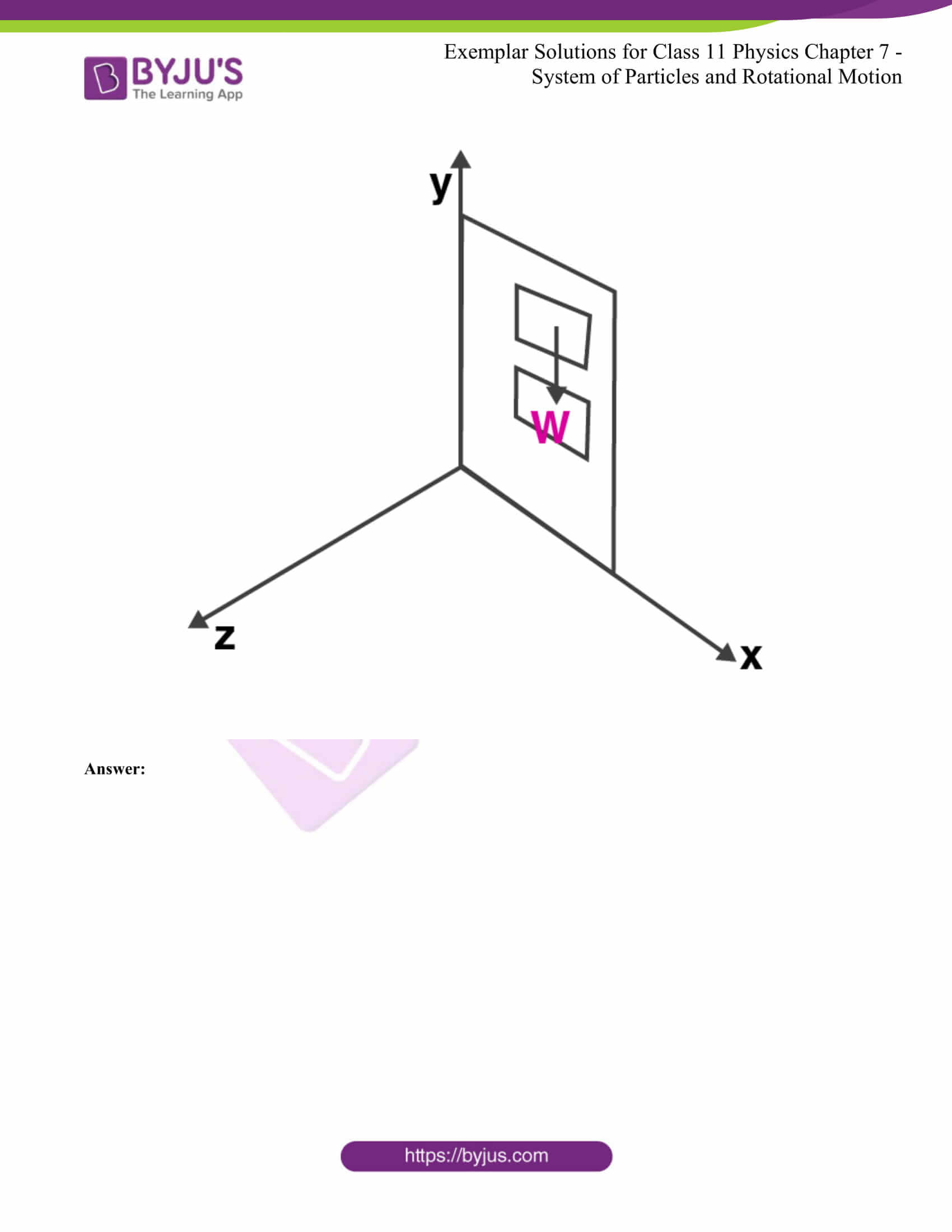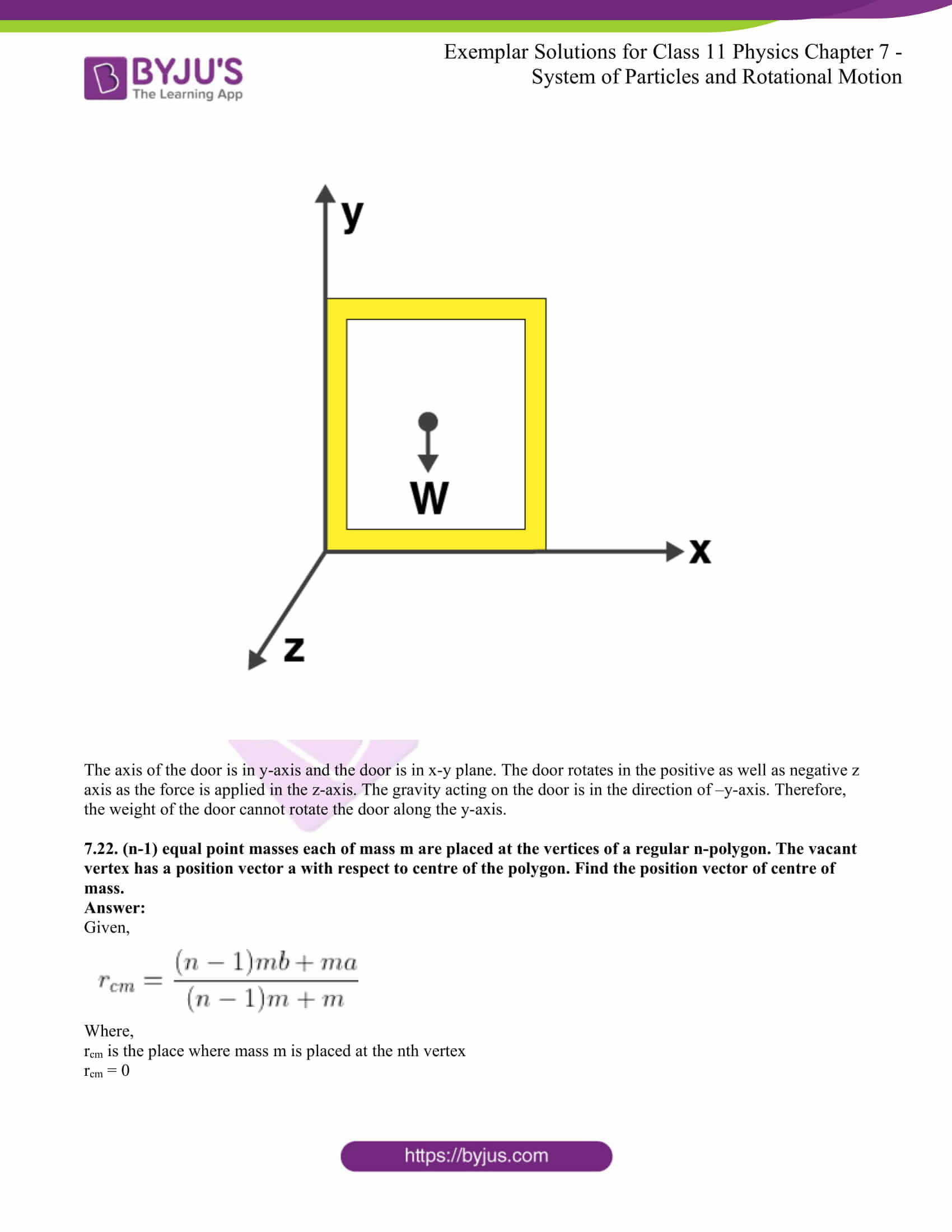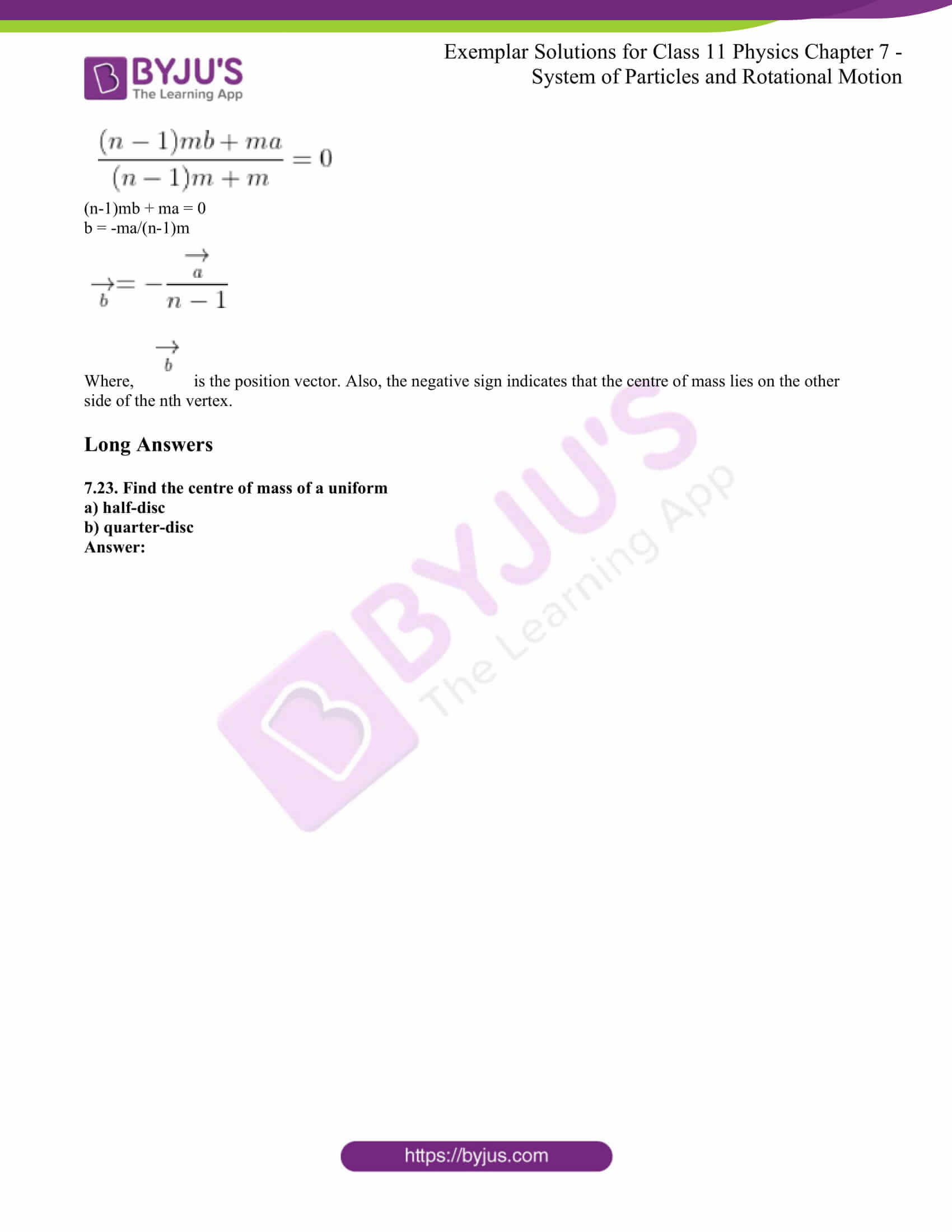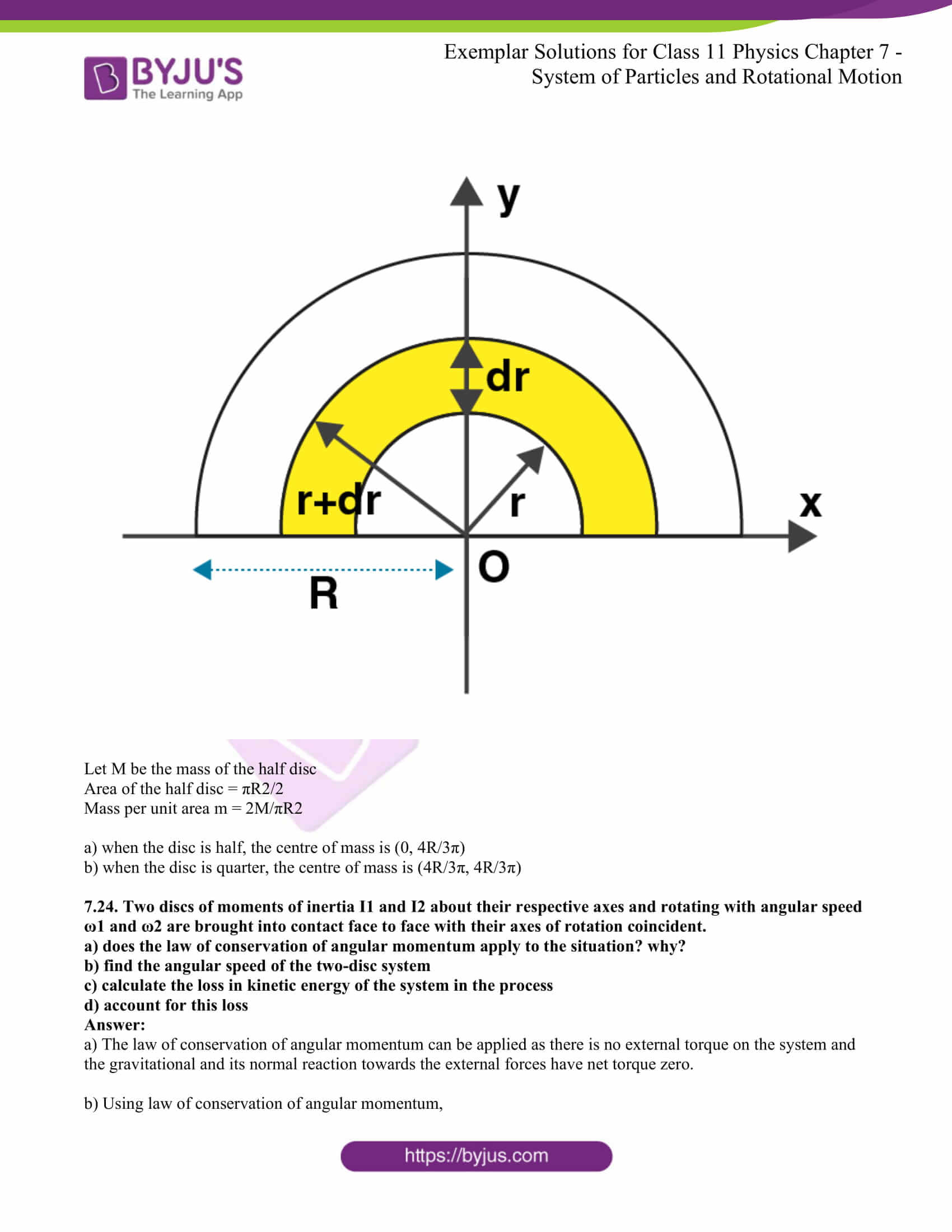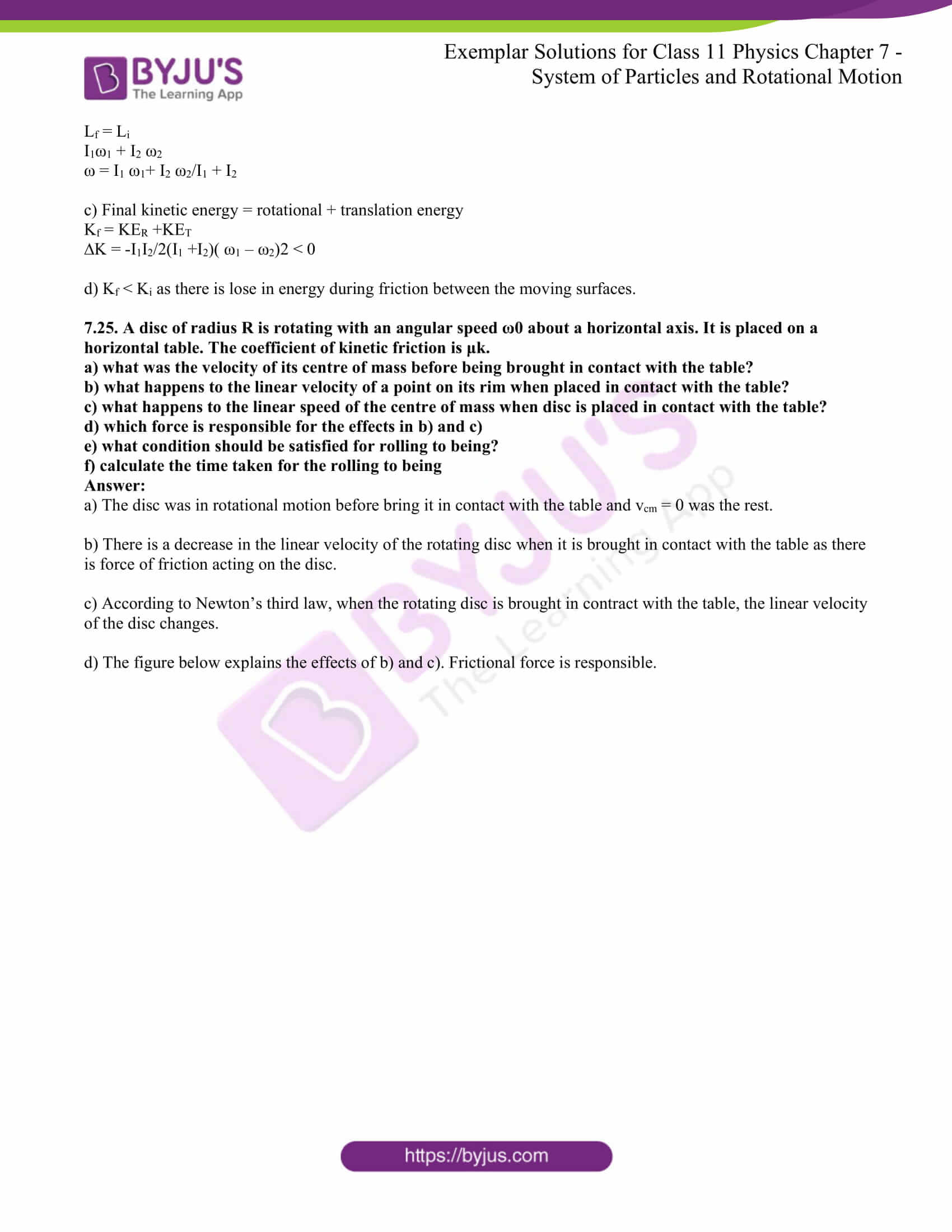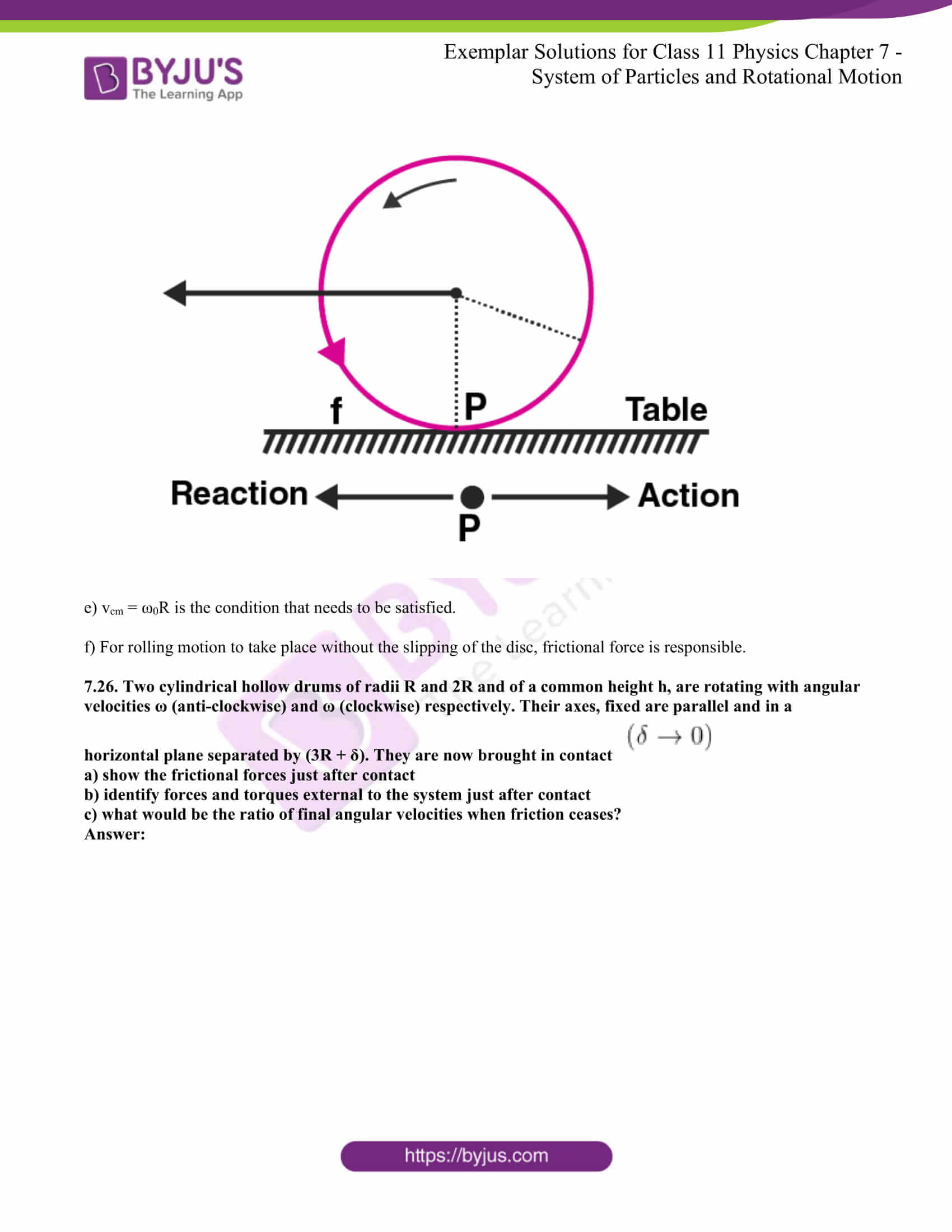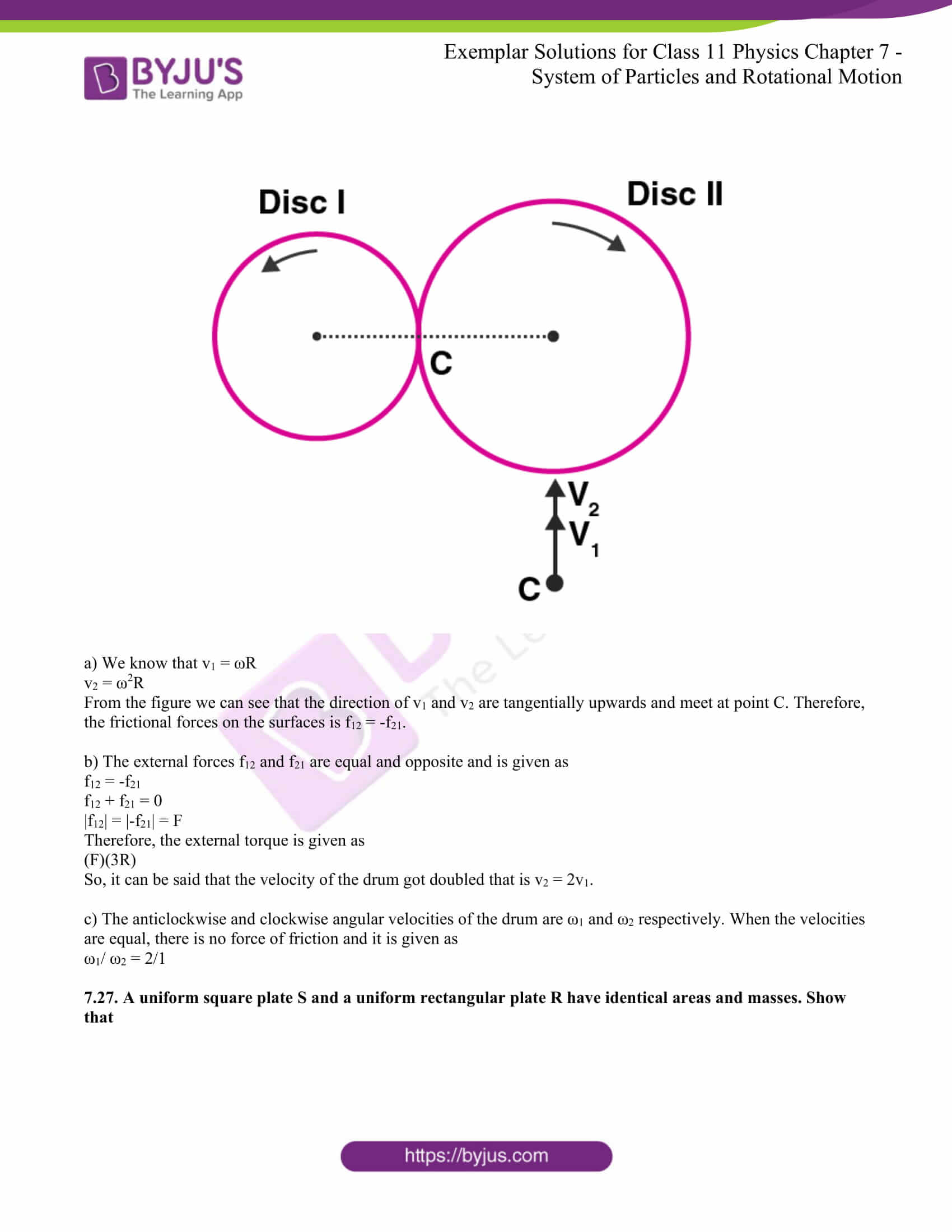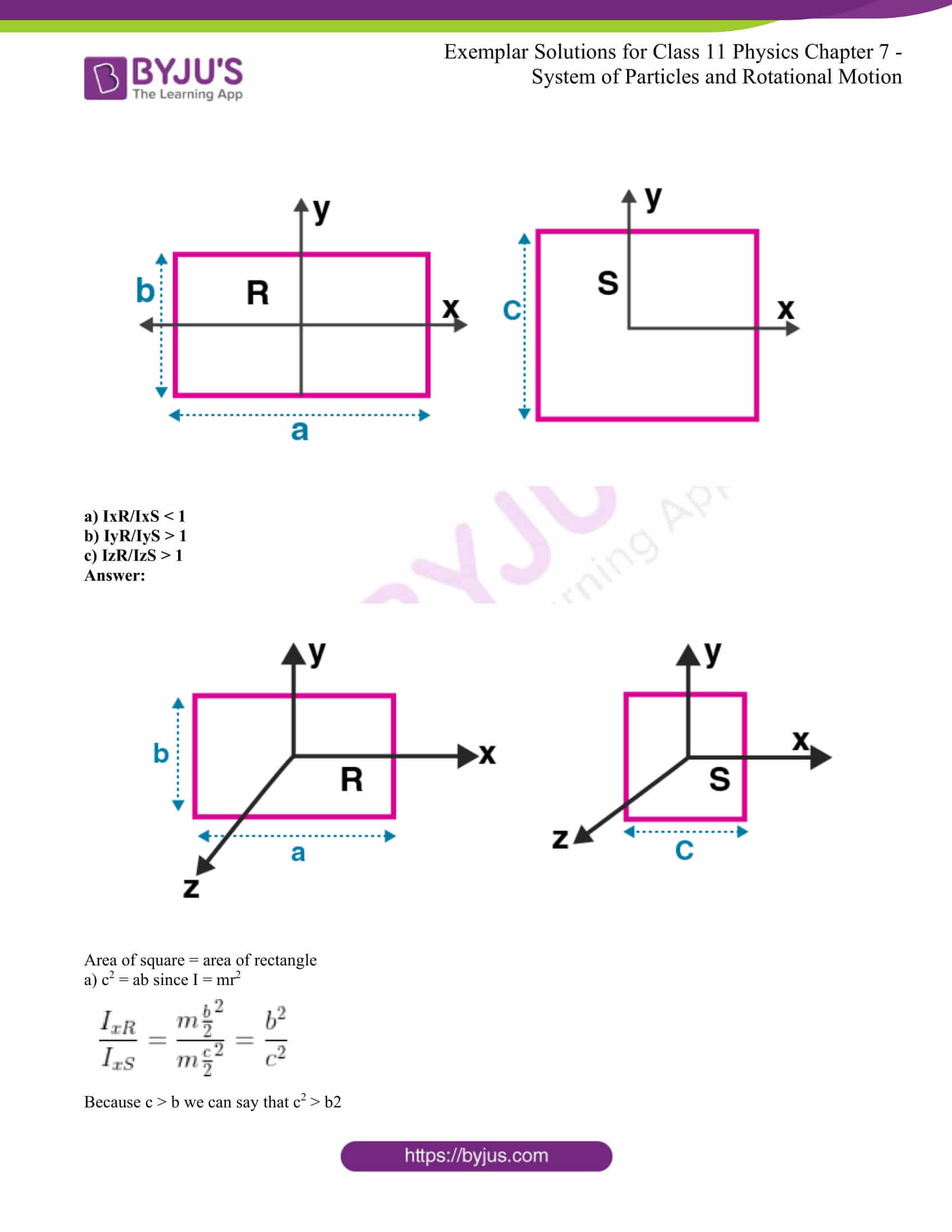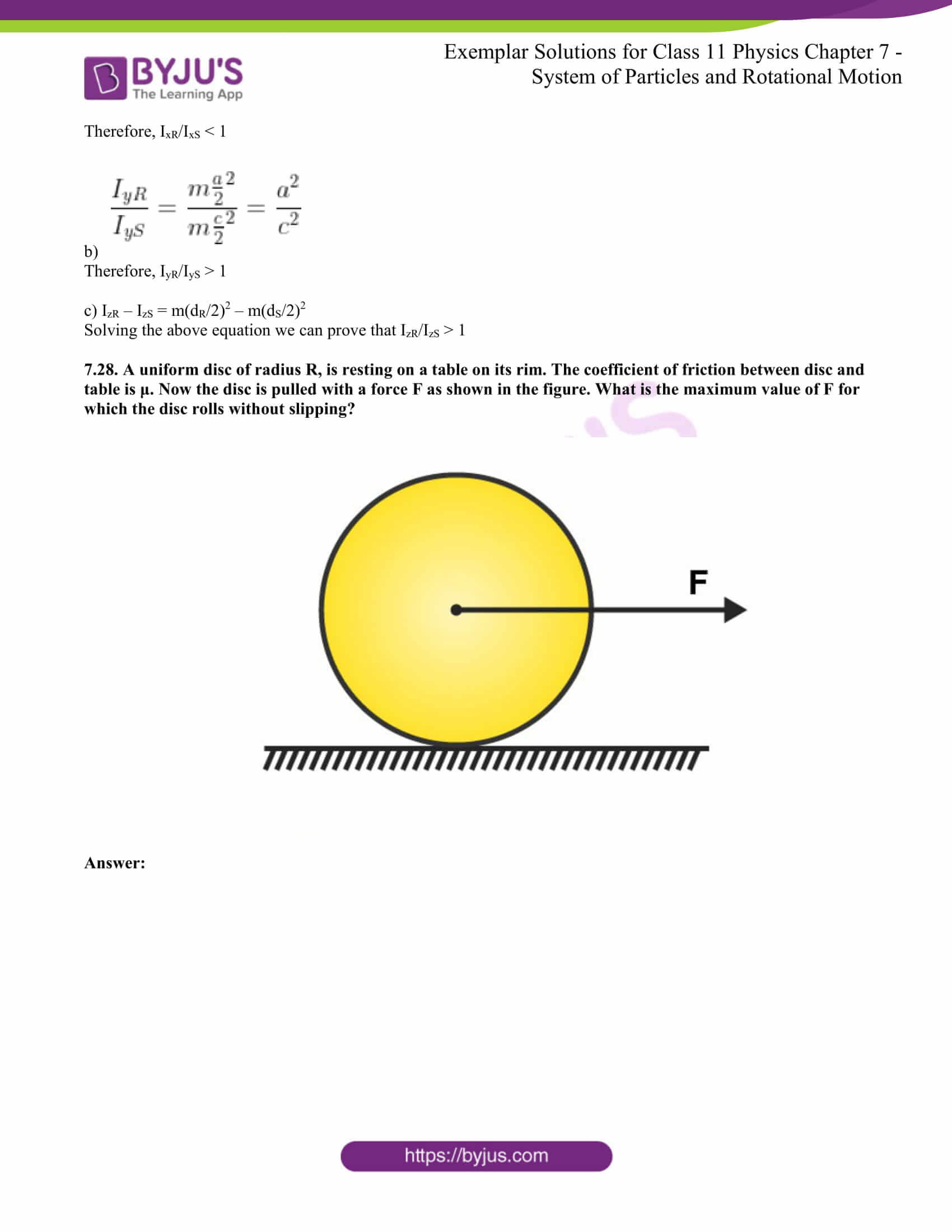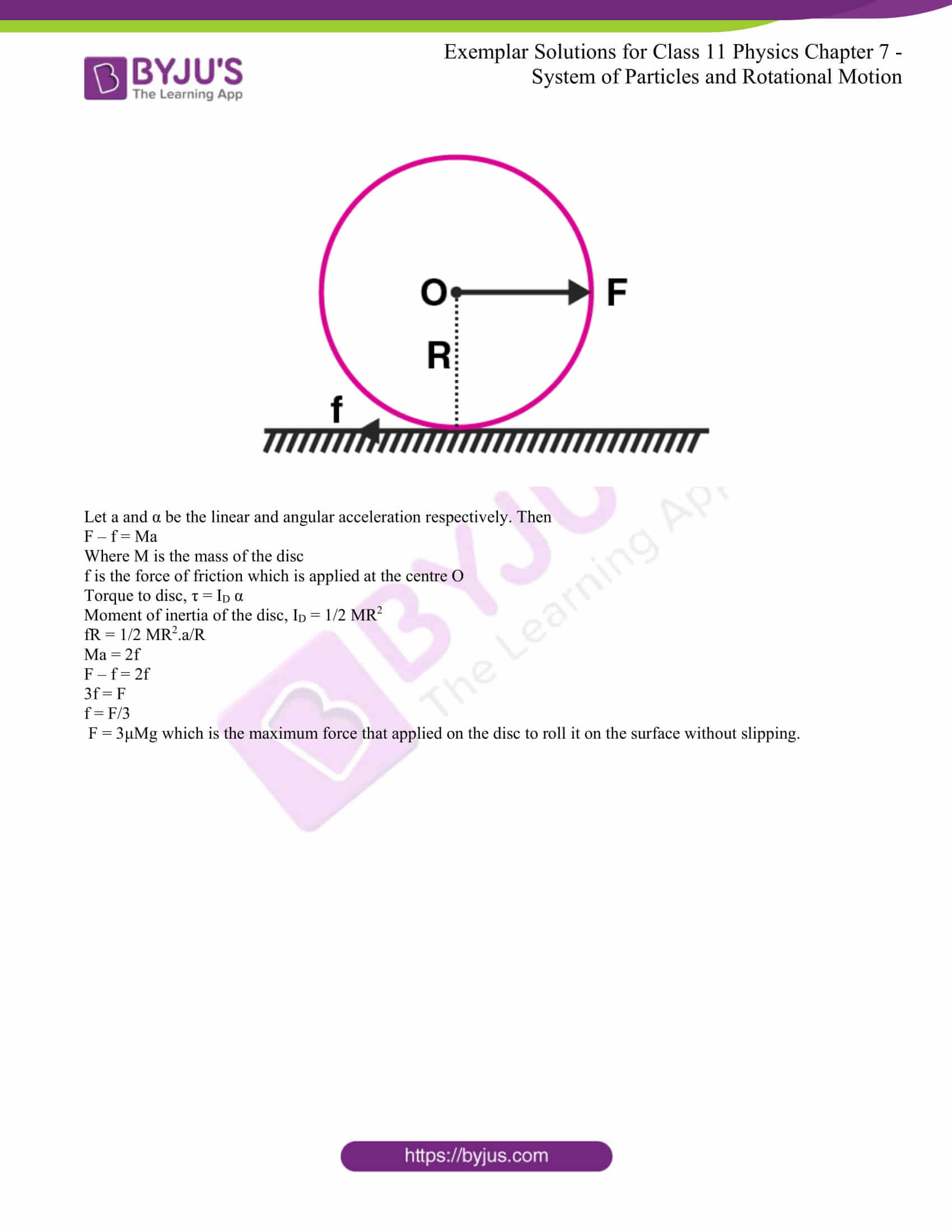### Multiple Choice Questions I

7.1. For which of the following does the centre of mass lie outside the body?

a) a pencil

b) a shotput

c) a dice

d) a bangle

The correct answer is d) a bangle

7.2. Which of the following points is the likely position of the centre of mass of the system shown in the figure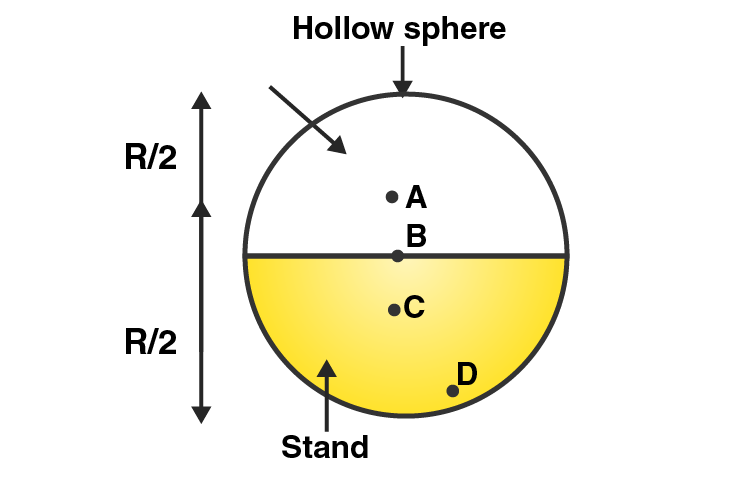a) A

b) B

c) C

d) D

The correct answer is c) C

7.3. A particle of mass m is moving in yz-plane with a uniform velocity v with its trajectory running parallel to +ve y-axis and intersecting z-axis at z = a. The change in its angular momentum about the origin as it bounces elastically from a wall at y = constant is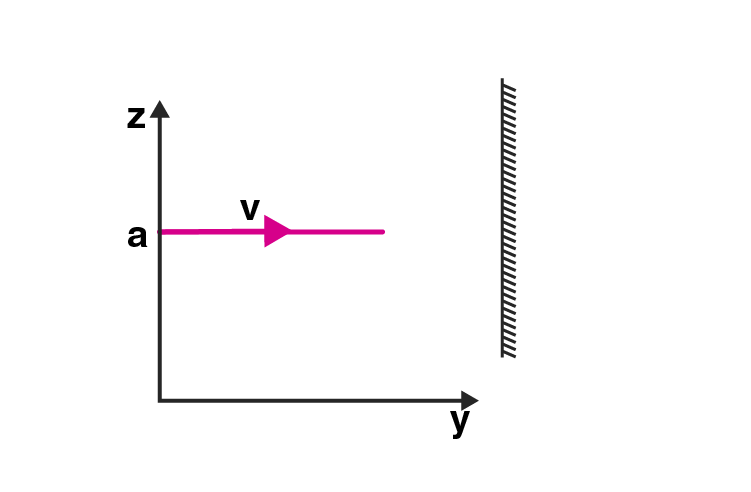a)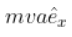b)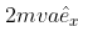c)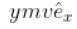d)7.4. When a disc rotates with uniform angular velocity, which of the following is not true?

a) the sense of rotation remains the same

b) the orientation of the axis of rotation remains the same

c) the speed of rotation is non-zero and remains the same

d) the angular acceleration is non-zero and remains the same

The correct answer is d) the angular acceleration is non-zero and remains same

7.5. A uniform square plate has a small piece Q of an irregular shape removed and glued to the centre of the plate leaving a hole behind. The moment of inertia about the z-axis is then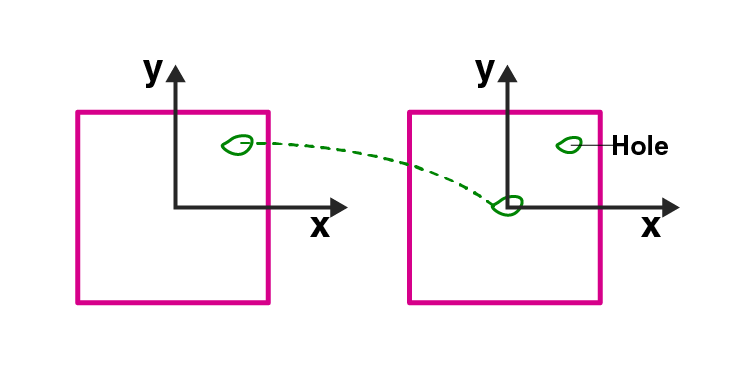a) increased

b) decreased

c) the same

d) changed in an unpredicted manner

The correct answer is b) decreased

7.6. In problem 7.5. the CM of the plate is now in the following quadrant of the x-y plane

a) I

b) II

c) III

d) IV

The correct answer is c) III

7.7. The density of a non-uniform rod of length 1 m is given by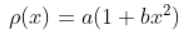where a and b are constant and 0 ≤ x ≤ 1. The centre of mass of the rod will be at

a)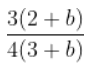b)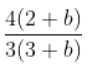c)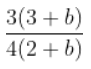d)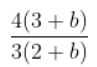7.8. A merry-go-round, made of a ring-like platform of radius R and mass M, is revolving with angular speed ω. A person of mass M is standing on it. At one instant, the person jumps off the ground, radially away from the centre of the round. The speed of the round afterwards is

a) 2ω

b) ω

c) ω/2

d) 0

The correct answer is a) 2ω

### Multiple Choice Questions II

7.9. Choose the correct alternatives:

a) for a general rotational motion, angular momentum L and angular velocity ω need not be parallel

b) for a rotational motion about a fixed axis, angular momentum L and angular velocity ω are always parallel

c) for a general translational motion, momentum p and velocity v is always parallel

d) for a general translational motion, acceleration a and velocity v are always parallel

a) for a general rotational motion, angular momentum L and angular velocity ω need not be parallel

c) for a general translational motion, momentum p and velocity v is always parallel

7.10. Figure shows two identical particles 1 and 2, each of mass m, moving in opposite directions with the same speed v along parallel lines. At a particular instant, r1 and r2 are their respective positions vectors drawn from point A which is in the plane of the parallel lines. Choose the correct options: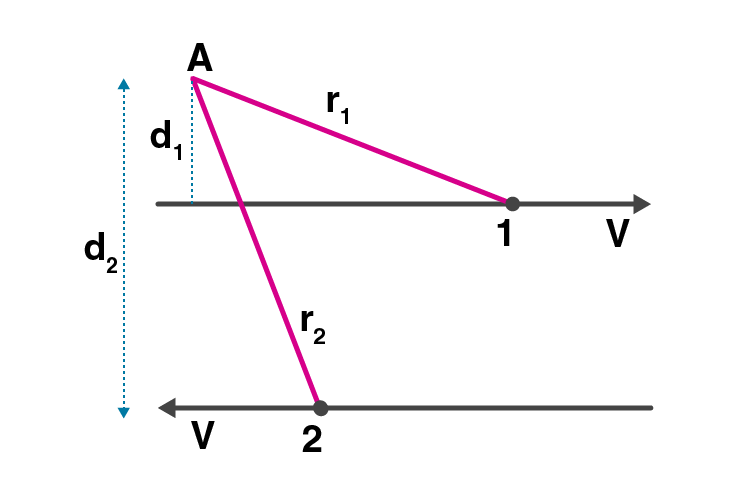a) angular momentum l1 of particle 1 is about A is l1 = mvd1

b) angular momentum l2 of particle 2 about A is l2 = mvx2

c) total angular momentum of the system about A is l = mv(r1 + r2)

d) total angular momentum of the system about A is l = mv(d2 – d1)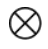represents a unit vector coming out of the page.a) angular momentum l1 of particle 1 is about A is l1 = mvd1

b) angular momentum l2 of particle 2 about A is l2 = mvx2

7.11. The net external torque on a system of particle about any axis is zero. Which of the following are compatible with it?

a) the forces may be acting radially from a point on the axis

b) the forces may be acting on the axis of rotation

c) the forces may be acting parallel to the axis of rotation

d) the torque caused by some forces may be equal and opposite to that caused by other forces

The correct is all the four options

7.12. Figure shows a lamina in the x-y plane. Two axes z and z’ pass perpendicular to its plane. A force F acts in the plane of lamina at point P as shown. Which of the following are true?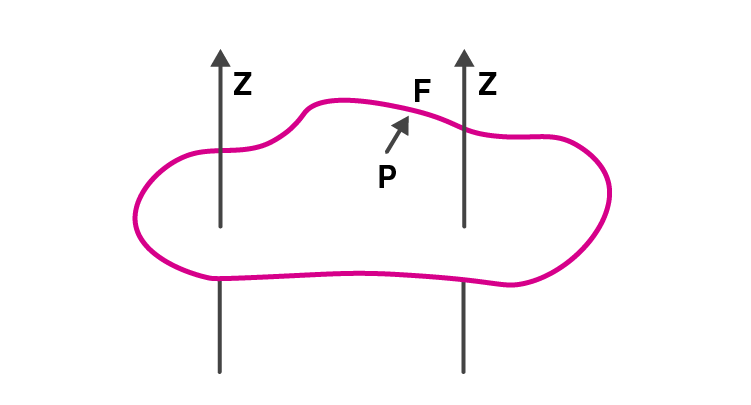a) torque τ caused by F about z-axis is along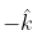b) torque τ’ caused by F about z’ axis is alongc) torque τ caused by F about the z-axis is greater in magnitude than that about the z-axis

d) total torque is given be τ = τ + τ’

b) torque τ’ caused by F about z’ axis is alongc) torque τ caused by F about the z-axis is greater in magnitude than that about the z-axis

7.13. Regarding figure, of a cube of edge a and mass n, state whether the following are true or false.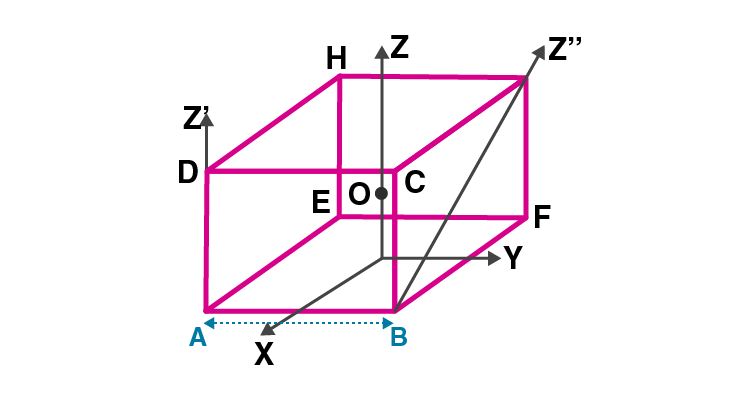a) the moment of inertia of cube about the z-axis is Iz – Ix + Iy

b) the moment of inertia of cube about z’ is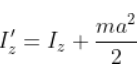c) the moment of inertia of cube about z’’ is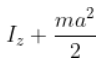d) Ix = Iy

b) the moment of inertia of cube about z’ isd) Ix = Iy

7.14. The centre of gravity of a body on the earth coincides with its centre of mass for a ‘small’ object whereas for an ‘extended’ object it may not. What is the qualitative meaning of ‘small’ and ‘extended’ in this regard? For which of the following the two coincides? A building, a pond, a lake, a mountain?

The centre of gravity is the geometric centre whereas the centre of mass is the mass of the point where the entire mass of the body is considered. The object is said to be small when the vertical height of the object is close to the earth’s surface. If the distance between the object and the surface of the earth is large then the object is extended object.

Building and pond are considered to be small objects while a mountain and a lake are considered to be extended objects.

7.15. Why does a solid sphere have a smaller moment of inertia than a hollow cylinder of same mass and radius, about an axis passing through their axes of symmetry?

The moment of inertia is directly proportional to the square of the distance of the mass from the axis of the rotation. In a solid sphere, the distribution of mass takes place from the centre to the radius of the sphere. Whereas in a hollow cylinder, the mass is concentrated on the peripheral surface. Therefore, the moment of inertia of the hollow cylinder is more than the moment of inertia of the cylinder.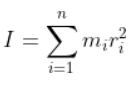7.16. The variation of angular position θ, of a point on a rotating rigid body, with time t is shown in figure. Is the body rotating clock-wise or anti-clockwise?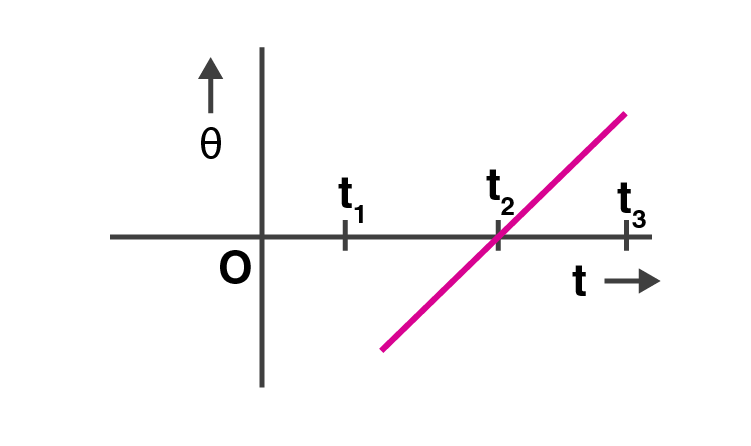The slope of the given graph is positive, therefore the rotation is clockwise.

dθ/dt = ω

7.17. A uniform cube of mass m and side a is placed in a frictionless horizontal surface. A vertical force F is applied to the edge as shown in the figure.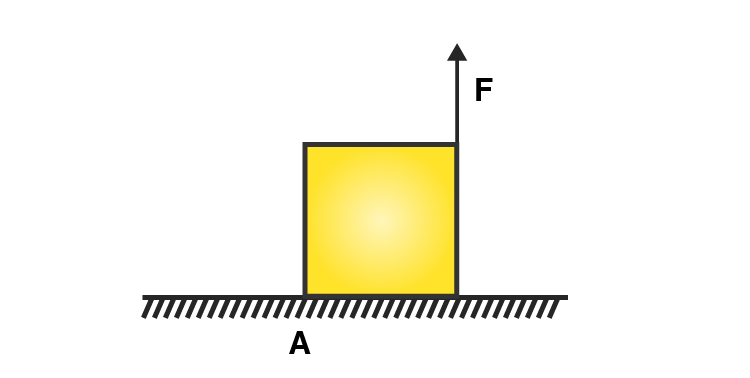Match the following

 a) mg/4 < F < mg/2 i) cube will move up b) F > mg/2 ii) cube will not exhibit motion c) F > mg iii) cube will begin to rotate and slip at A d) F = mg/4 iv) normal reaction effectively at a/3 from A, no motion

a) matches with ii)

b) matches with iii)

c) matches with i)

d) matches with iv)

7.18. A uniform sphere of mass m and radius R is placed on a rough horizontal surface. The sphere is struck horizontally at a height h from the floor.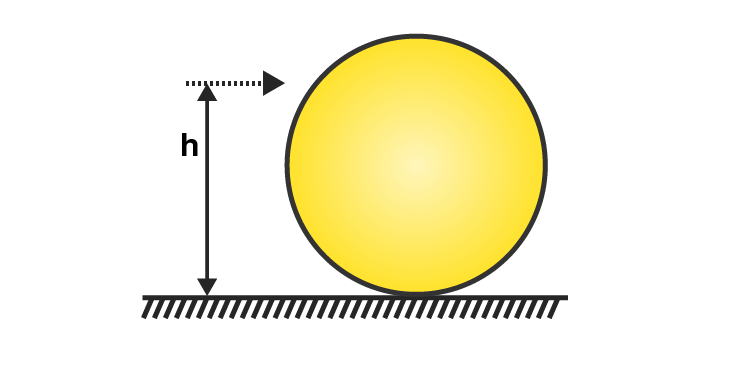Match the following

 a) h = R/2 i) sphere rolls without slipping with a constant velocity and no loss of energy b) h = R ii) sphere spins clockwise, loses energy by friction c) h = 3R/2 iii) sphere spins anti-clockwise, loses energy by friction d) h = 7R/5 iv) sphere has only a translational motion, loses energy by friction

a) matches with iii)

b) matches with iv)

c) matches with ii)

d) matches with i)

7.19. The vector sum of a system of non-collinear forces acting on a rigid body is given to be non-zero. If the vector sum of all the torques due to the system of forces about a certain point is found to be zero, does this mean that it is necessarily zero about any arbitrary point?

The vector sum of the torques is zero. But the resultant force is not zero. The mathematical explanation is given as: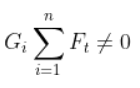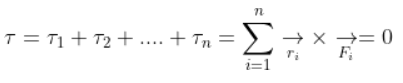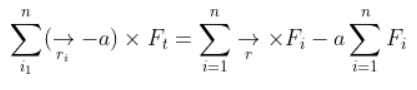7.20. A wheel in uniform motion about an axis passing through its centre and perpendicular to its plane is considered to be in mechanical equilibrium because no net external force or torque is required to sustain its motion. However, the particles that constitute the wheel do experience a centripetal acceleration directed towards the centre. How do you reconcile this fact with the wheel being in equilibrium? How would you set a half-wheel into uniform motion about an axis passing through the centre of mass of the wheel and perpendicular to its plane? Will you require external forces to sustain the motion?

A wheel is a rigid elastic body with a uniform motion which is passing through its centre and is perpendicular to the plane of the wheel. Every particle of the wheel experiences a centripetal acceleration and is directed towards the axis of rotation due to elastic force. When the wheel is half, the distribution of the mass also becomes half. When the mass is half, there is no symmetry in the wheel. Therefore, the angular momentum and angular velocity does not coincide. Therefore, the external torque is required.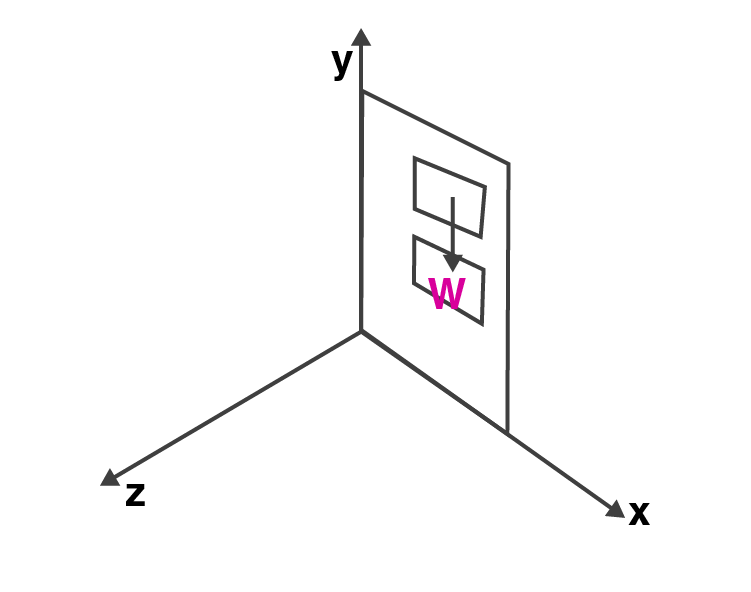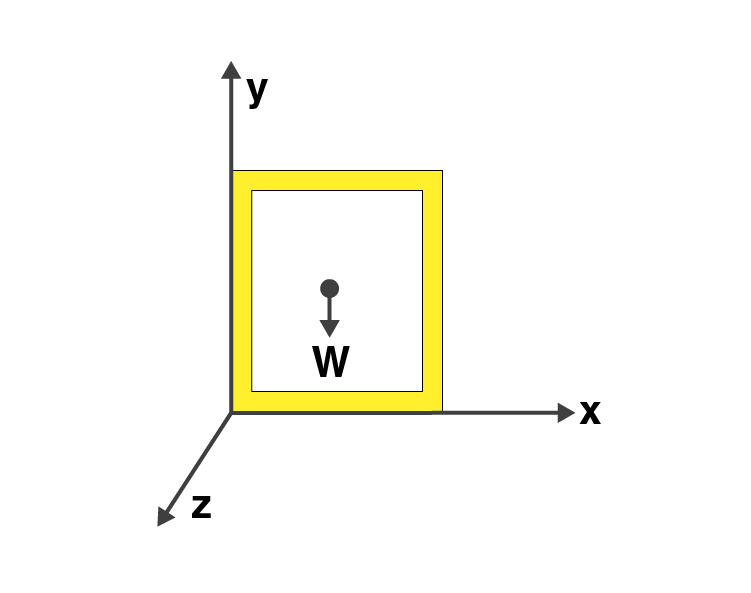The axis of the door is in the y-axis and the door is in the x-y plane. The door rotates in the positive as well as negative z-axis as the force is applied in the z-axis. The gravity acting on the door is in the direction of –y-axis. Therefore, the weight of the door cannot rotate the door along the y-axis.

7.22. (n-1) equal point masses each of mass m are placed at the vertices of a regular n-polygon. The vacant vertex has a position vector concerning the centre of the polygon. Find the position vector of the centre of mass.

Given,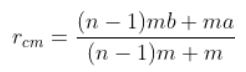Where,

rcm is the place where mass m is placed at the nth vertex

rcm = 0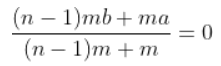(n-1)mb + ma = 0

b = -ma/(n-1)m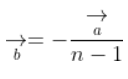Where,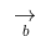is the position vector. Also, the negative sign indicates that the centre of mass lies on the other side of the nth vertex.

7.23. Find the centre of mass of a uniform

a) half-disc

b) quarter-disc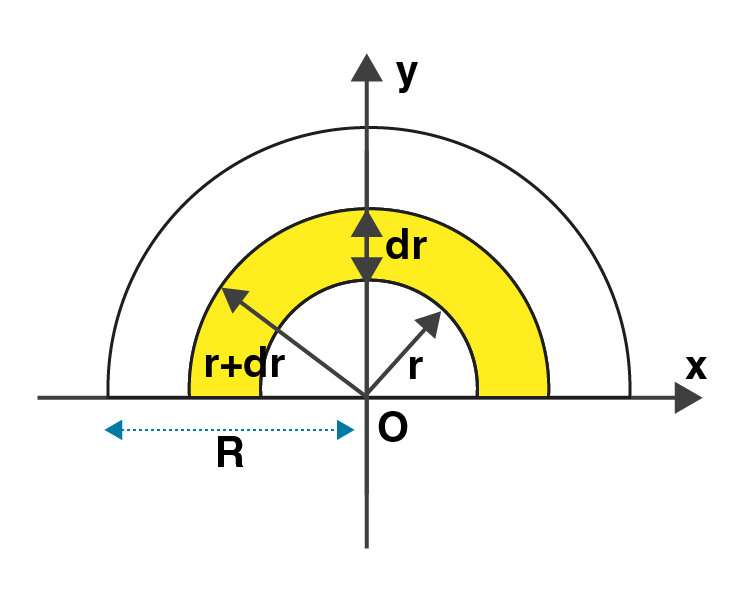Let M be the mass of the half-disc

Area of the half-disc = πR2/2

Mass per unit area m = 2M/πR2

a) when the disc is half, the centre of mass is (0, 4R/3π)

b) when the disc is quarter, the centre of mass is (4R/3π, 4R/3π)

7.24. Two discs of moments of inertia I1 and I2 about their respective axes and rotating with angular speed ω1 and ω2 are brought into contact face to face with their axes of rotation coincident.

a) does the law of conservation of angular momentum apply to the situation? why?

b) find the angular speed of the two-disc system

c) calculate the loss in kinetic energy of the system in the process

d) account for this loss

a) The law of conservation of angular momentum can be applied as there is no external torque on the system and the gravitational and its normal reaction towards the external forces have net torque zero.

b) Using law of conservation of angular momentum,

Lf = Li

I1ω1 + I2 ω2

ω = I1 ω1+ I2 ω2/I1 + I2

c) Final kinetic energy = rotational + translation energy

Kf = KER +KET

∆K = -I1I2/2(I1 +I2)( ω1 – ω2)2 < 0

d) Kf < Ki as there is lose in energy during friction between the moving surfaces.

7.25. A disc of radius R is rotating with an angular speed ω0 about a horizontal axis. It is placed on a horizontal table. The coefficient of kinetic friction is μk.

a) what was the velocity of its centre of mass before being brought in contact with the table?

b) what happens to the linear velocity of a point on its rim when placed in contact with the table?

c) what happens to the linear speed of the centre of mass when the disc is placed in contact with the table?

d) which force is responsible for the effects in b) and c)

e) what condition should be satisfied for rolling to being?

f) calculate the time taken for the rolling to being

a) The disc was in rotational motion before bringing it in contact with the table and vcm = 0 was the rest.

b) There is a decrease in the linear velocity of the rotating disc when it is brought in contact with the table as there is a force of friction acting on the disc.

c) According to Newton’s third law, when the rotating disc is brought in contract with the table, the linear velocity of the disc changes.

d) The figure below explains the effects of b) and c). Frictional force is responsible.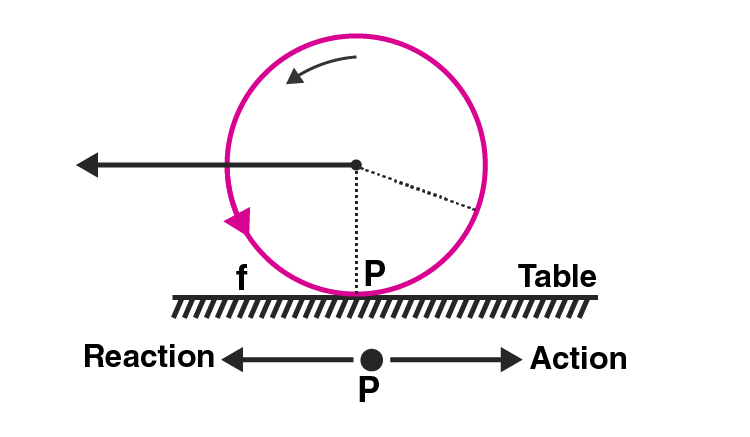e) vcm = ω0R is the condition that needs to be satisfied.

f) For rolling motion to take place without the slipping of the disc, the frictional force is responsible.

7.26. Two cylindrical hollow drums of radii R and 2R and of a common height h, are rotating with angular velocities ω (anti-clockwise) and ω (clockwise) respectively. Their axes, fixed are parallel and in a horizontal plane separated by (3R + δ). They are now brought in contact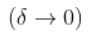a) show the frictional forces just after contact

b) identify forces and torques external to the system just after contact

c) what would be the ratio of final angular velocities when friction ceases?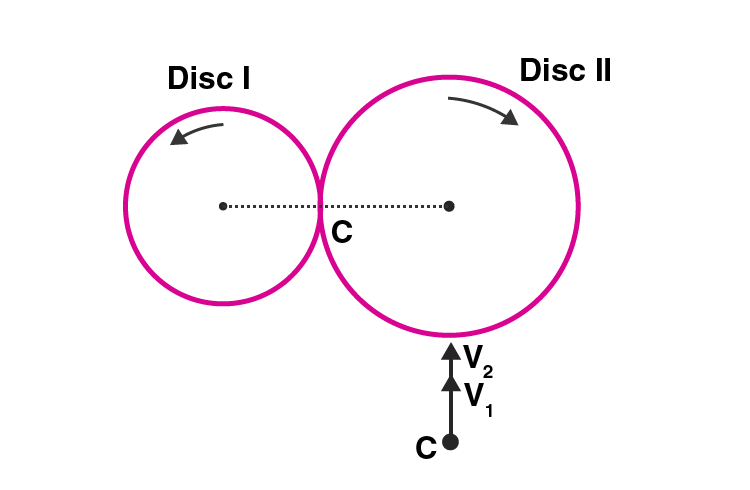a) We know that v1 = ωR

v2 = ω2R

From the figure we can see that the direction of v1 and v2 are tangentially upwards and meet at point C. Therefore, the frictional forces on the surfaces is f12 = -f21.

b) The external forces f12 and f21 are equal and opposite and is given as

f12 = -f21

f12 + f21 = 0

|f12| = |-f21| = F

Therefore, the external torque is given as

(F)(3R)

So, it can be said that the velocity of the drum got doubled that is v2 = 2v1.

c) The anticlockwise and clockwise angular velocities of the drum are ω1 and ω2 respectively. When the velocities are equal, there is no force of friction and it is given as

ω1/ ω2 = 2/1

7.27. A uniform square plate S and a uniform rectangular plate R have identical areas and masses. Show that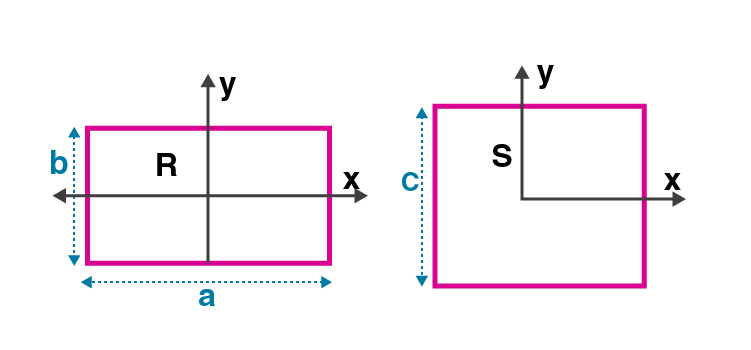a) IxR/IxS < 1

b) IyR/IyS > 1

c) IzR/IzS > 1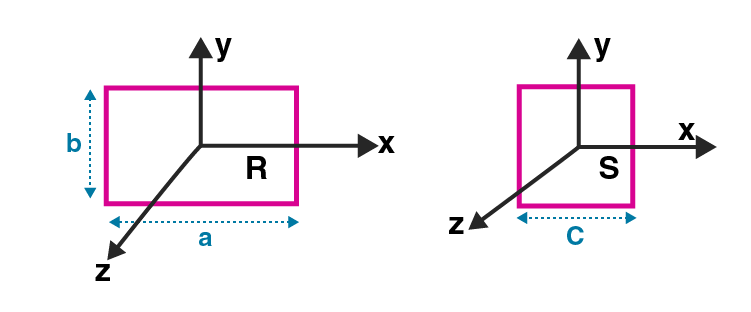Area of square = area of rectangle

a) c2 = ab since I = mr2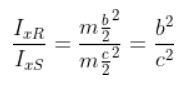Because c > b we can say that c2 > b2

Therefore, IxR/IxS < 1

b)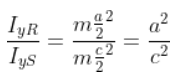Therefore, IyR/IyS > 1

c) IzR – IzS = m(dR/2)2 – m(dS/2)2

Solving the above equation we can prove that IzR/IzS > 1

7.28. A uniform disc of radius R, is resting on a table on its rim. The coefficient of friction between disc and table is μ. Now the disc is pulled with a force F as shown in the figure. What is the maximum value of F for which the disc rolls without slipping?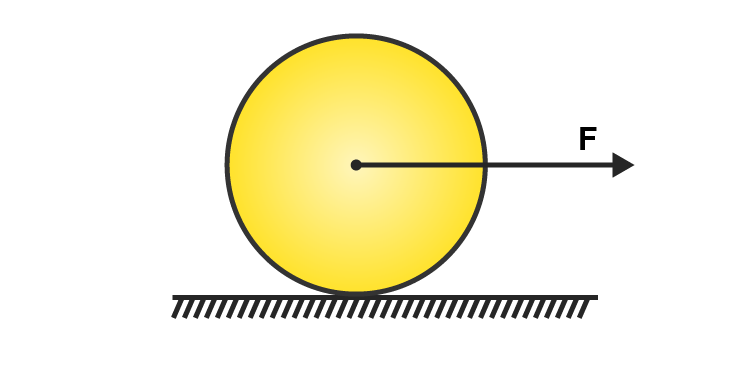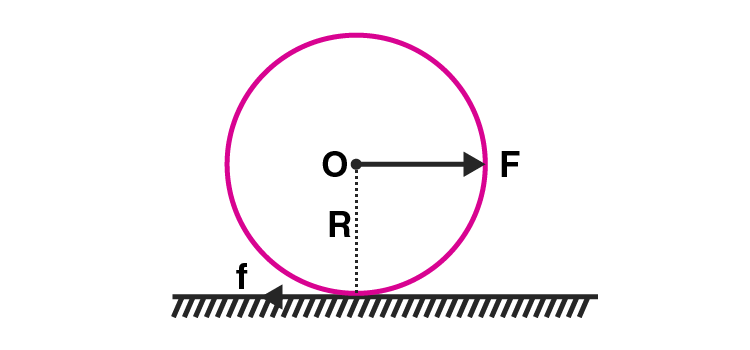Let a and α be the linear and angular acceleration respectively. Then

F – f = Ma

Where M is the mass of the disc

f is the force of friction which is applied at the centre O

Torque to disc, τ = ID α

Moment of inertia of the disc, ID = 1/2 MR2

fR = 1/2 MR2.a/R

Ma = 2f

F – f = 2f

3f = F

f = F/3

F = 3μMg which is the maximum force that applied on the disc to roll it on the surface without slipping.

### Why Opt For BYJU’S?

BYJU’S believe in creating world-class learning material for the students. Our study material boosts their creativity, problem-solving skills and thinking pattern. Apart from high-quality learning content we also believe that students should be supported extensively in their education journey. Taking this into consideration, BYJU’S has some of the best subject experts who can guide students to learn the subject and its concepts in a more simple and conceptual manner. In order to help students complete and succeed in their journey, BYJU’S keeps a track of all the progress that student’s make and offers feedback on regular intervals after some assessment. Students can also contact our responsive support team to ask all their doubts on any subject of CBSE syllabus.